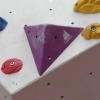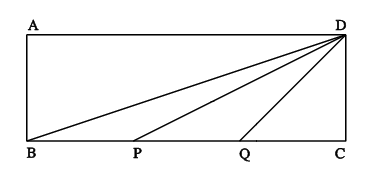#### You may also likeA quadrilateral changes shape with the edge lengths constant. Show the scalar product of the diagonals is constant. If the diagonals are perpendicular in one position are they always perpendicular?### Tetra Perp

Show that the edges AD and BC of a tetrahedron ABCD are mutually perpendicular when: AB²+CD² = AC²+BD².As a quadrilateral Q is deformed (keeping the edge lengths constnt) the diagonals and the angle X between them change. Prove that the area of Q is proportional to tanX.

# Three by One

##### Age 16 to 18Challenge Level

$ABCD$ is a rectangle where $BC$ = $3AB$. $P$ and $Q$ are points on $BC$ such that $BP$ = $PQ$ = $QC$.Show that: angle $DBC$ + angle $DPC$ = angle $DQC$

Generalise this result.

N.B. This problem can be tackled in at least 8 different ways using different mathematics learnt in the last two years in school and earlier. The methods are essentially the same when viewed from a more advanced perspective.